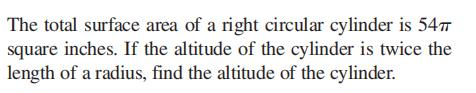### Still have math questions?

Algebra
Question$$\left. \begin{array} { l } { \text { The total surface area of a right circular cylinder is } 54 \pi } \\ { \text { square inches. If the altitude of the cylinder is twice the } } \\ { \text { length of a radius, find the altitude of the cylinder. } } \end{array} \right.$$

$$6 \text { inches }$$